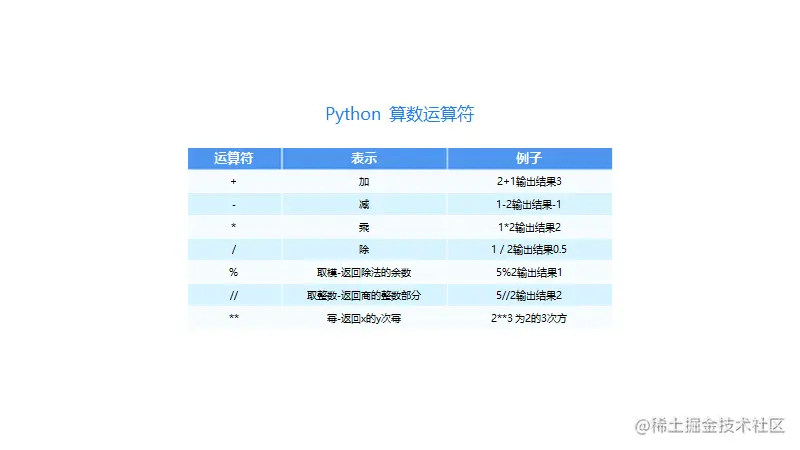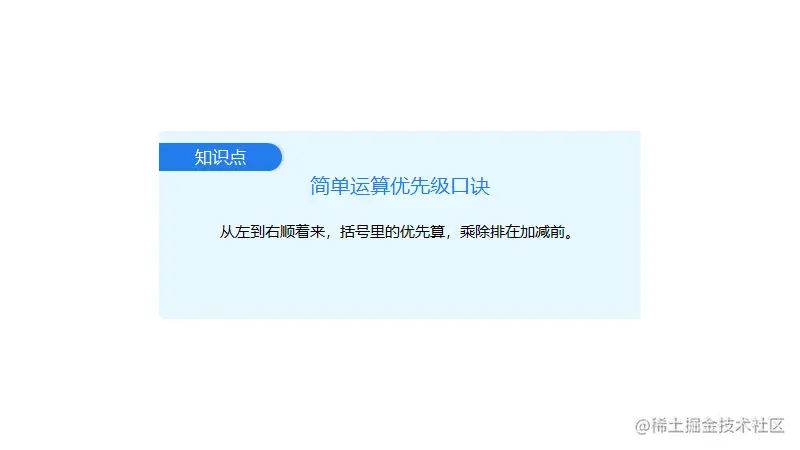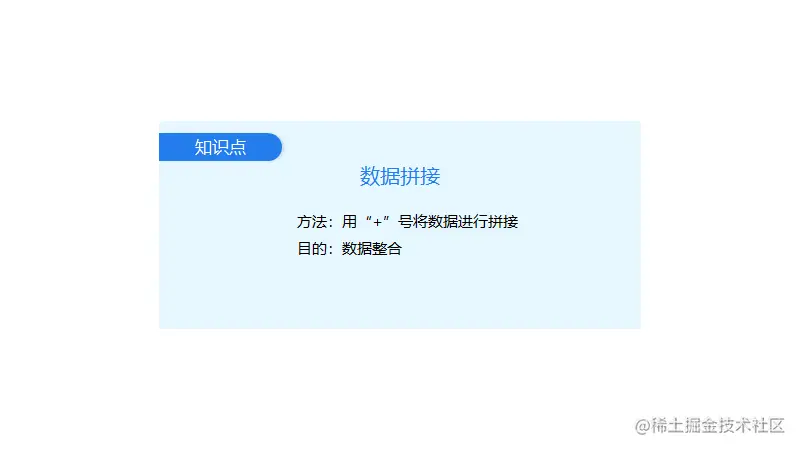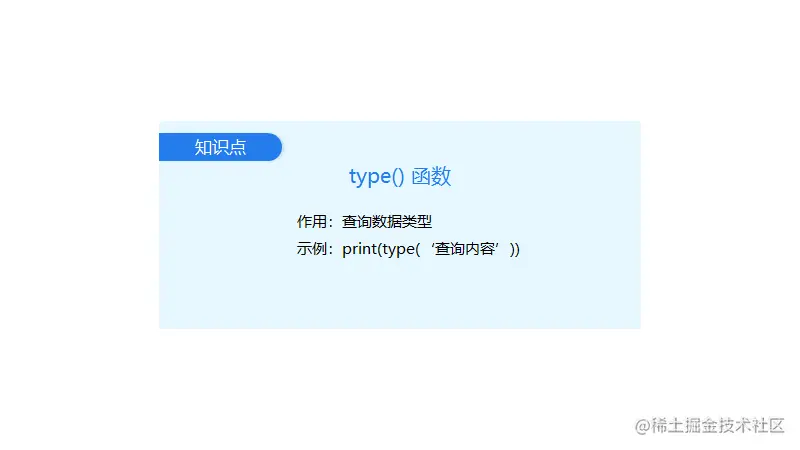# Python from 0 to 1 (day 11) - Python data application 1

2022-01-31 17:42:59

This is my participation 11 The fourth of the yuegengwen challenge 16 God , Check out the activity details ：2021 One last more challenge

### arithmetic

Python Integer in 、 Floating point numbers and integers in mathematical sense 、 Decimals are the same , Its most important function is to combine with operation symbols , Conduct Mathematical calculation . Let's take a look Python How to use integers , There are four operations on floating-point numbers .

however Python The arithmetic operators of the world , It's the same as the arithmetic symbols we usually write on paper , There are also differences .

First , Let's see Python Operators of the world .however , For so many arithmetic operators , I suggest you look at it first , Just have a general impression . You can save this picture first , I'll find it when I use it , The corresponding reference can be .

Let's talk about the same thing again —— Operation priority ：Python Operational priorities in the world , It's the same priority as our usual calculation .below , Please click the run button , Run through the code .

``````print(499*561+10620-365)

print((5025-525)/100+18*17)
Copy code ``````

Let's see 【print((5025-525)/100+18*17)】 The order of operations ： First calculate the... In parentheses 【5025-525】.

And then 【5025-525】 Divided by 100, The third step is to calculate 【18*17】, Finally, add the results of division and multiplication .

### String splicing

For example , I have the following fragmentary 【 words 】—— Variable , Then I want to synthesize such a sentence ： I want to buy a game console , cleanser , Instant noodles and microwave ovens , Authentication in the mirrored world , What are you going to do ？

``````hero = ' I '

action = ' I want to buy '

play = ' console '

wash = ' cleanser '

eat = ' instant noodles '

electric = ' The microwave oven '

#  Output results

#  I want to buy a game console , cleanser , Instant noodles and microwave ovens
Copy code ``````

This involves our next knowledge point —— String splicing .

The method of string splicing is simple , Is to use string splicing symbols 【+】, Connect the variables that need to be spliced together .

such as , The above example , I can write ：

``````hero = ' I '

action = ' I want to buy '

play = ' console '

wash = ' cleanser '

eat = ' instant noodles '

electric = ' The microwave oven '

print(hero + action + play + ',' + wash + ',' + eat + ' and ' + electric)
Copy code ``````

With the splicing symbol , I can not only output 【 I want to buy a game console , cleanser , Instant noodles and microwave ovens 】, You can also adjust the splicing order of variables , Output different content . Learned string splicing , Can make our shopping more flexible , Combine whatever we want to buy .

This is us String splicing Some key points ：## Query of data type ——`type()` function

that , This `type()` How to use the function ？ The answer is ： Just put the contents of the query in brackets .As long as you put the content in parentheses ,`type()` Function will immediately find out its type . below , Please try the code just now .

``````who = ' I '

action = ' To be in '

destination = ' Meituan takeout '

how = ' spot '

number = 10

what = ' Fried chicken '

print(type(who))

print(type(action))

print(type(destionation))

print(type(how))

print(type(number))

print(type(what))
Copy code ``````

The result in the terminal , Except for 5 individual `<class 'str'>`, also 1 individual `<class 'int'>`？ But why can't different types of data be spliced together ？ In a word ： Different circles don't blend .

Let me make an analogy , It's similar to me , You're at Tottenham . Although we are both football fans , But the team you like is the enemy , So there's no way to make friends , Can't put us together .

## summary

Python Integer in 、 Floating point numbers and integers in mathematical sense 、 Decimals are the same , Its most important function is to combine with operation symbols , Conduct Mathematical calculation . Let's take a look Python How to use integers , There are four operations on floating-point numbers .

The method of string splicing is simple , Is to use string splicing symbols 【+】, Connect the variables that need to be spliced together .

Be careful String splicing is not allowed for different data types ~